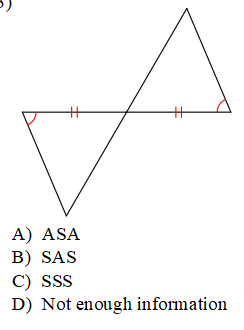Section 3 Skills Maint 4
Use the congruent statement to determine what segment is congruent to CB *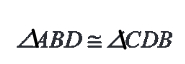Last Name *
Determine if these two triangles are congruent. If so, State the method **Find the marked angle *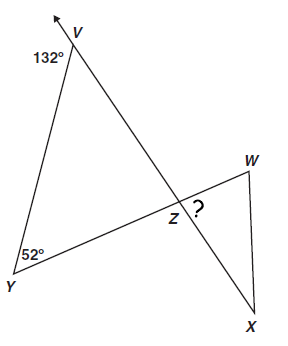*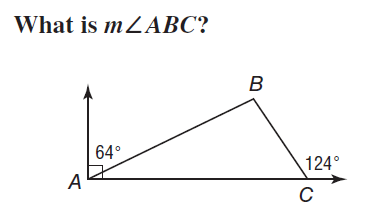Find the identified angle *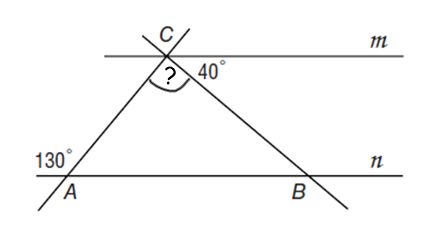Find angle in this isosceles triangle *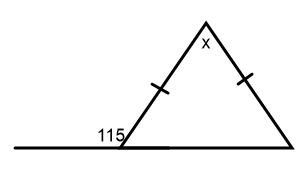*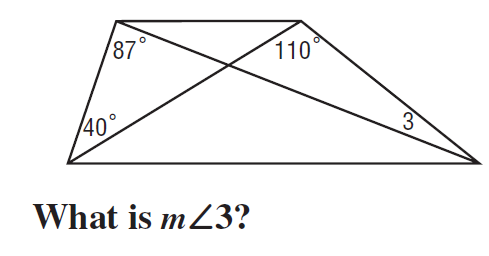One acute angle of a right triangle is 38 degrees. What is the measure of the other acute angle? *
One base angle of an isosceles triangle is 30 degrees. What is the measure of the vertex angle? *
Solve for x. *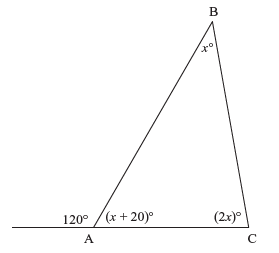Period *
*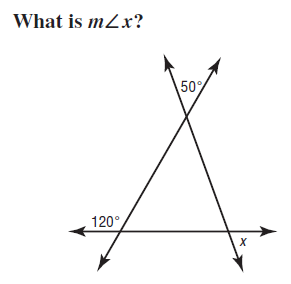Which of the following methods is not enough to say two triangles are congruent? *
Determine if these two triangles are congruent. If so, State the method *